HLIBpro  2.3.1
Boundary Element Methods

In this example, an integral equation is to be solved by representing the discretised operator with an 𝓗-matrix, whereby the 𝓗-matrix is constructed using a matrix coefficient function for entries of the equation system. Furthermore, for the iterative solver a preconditioner is computed using 𝓗-LU factorisation.

# Problem Description

Given the integral equation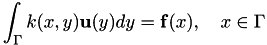with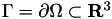and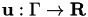being sought for a given right hand side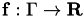, the Galerkin discretisation with ansatz functions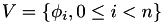and test functions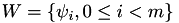leads to a linear equation system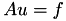where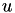contains the coefficients of the discretised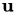and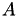is defined by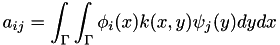The right hand side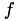is given by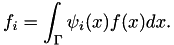# Surface Grid and Function Spaces

The code starts with the standard initialisation:

#include <iostream>
#include <hlib.hh>
using namespace HLIB;
using namespace std;
int main ( int argc, char ** argv )
{
try
{
INIT();

𝓗𝖫𝖨𝖡𝗉𝗋𝗈 supports triangular surface grids and can load such grids stored in several file formats, e.g. HLIB, PLY, SurfaceMesh and Gmsh v2 format. Although individual I/O classes for each file format exist, you may also use automatic file format detection:

auto grid = read_grid( "grid.tri" );

Based upon the grid, function spaces for the ansatz and the test space are defined. For Laplace and Helmholtz kernels, piecewise constant and linear function spaces are available, whereas for Maxwell kernels, piecewise constant edge space (RWG elements) is implemented.

auto ansatz_fnspace = make_unique< TLinearFnSpace >( grid.get() );
auto test_fnspace = make_unique< TLinearFnSpace >( grid.get() );
Remarks
Different grids may be used for ansatz and test spaces.

The functions spaces provide necessary geometrical information for construction cluster trees and block cluster trees for the defined index sets:

auto coord1 = ansatz_fnspace->build_coord();
auto coord2 = test_fnspace->build_coord();
TAutoBSPPartStrat part_strat;
TBSPCTBuilder ct_builder( & part_strat );
auto ct1 = ct_builder.build( coord1.get() );
auto ct2 = ct_builder.build( coord2.get() );
complex kappa( -2, 1 );
THiLoFreqGeomAdmCond adm_cond( kappa, 10 );
TBCBuilder bct_builder;
auto bct = bct_builder.build( ct1.get(), ct2.get(), & adm_cond );

Here, the admissibility condition THiLoFreqGeomAdmCond for oscillatory kernels was used, which tests if the given number of wave lengths (10) will fit into a given cluster to enable low-rank approximations. This is especially necessary if kappa is large.

# Definition of Kernel Function and Matrix Construction

In 𝓗𝖫𝖨𝖡𝗉𝗋𝗈, different kernels are defined by special bilinear forms, each derived from TBEMBF. For reasons of efficiency, e.g. for basis function evaluation, the ansatz spaces are provided to each bilinear form as template arguments. For the Helmholtz single layer potential, the corresponding bilinear form is declared as:

using slpbf_t = THelmholtzSLPBF< TLinearFnSpace, TLinearFnSpace >;
slpbf_t slpbf( kappa, ansatz_fnspace.get(), test_fnspace.get() );

Here, kappa is the wave number of the underlying problem.

Remarks
When template based classes are used, as here with the Helmholtz bilinear form, type aliases will increase readability of the code.

For matrix construction, the bilinear form is not fully sufficient as actual matrix coefficients are needed. These are provided by a special coefficient function:

TBFCoeffFn< slpbf_t > coeff_fn( & slpbf, bct->row_ct()->perm_i2e(), bct->col_ct()->perm_i2e() );

Finally, the low-rank approximation technique and the block-wise accuracy gave to be defined, being ACA+ and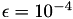in our case. Equipped with these, the TDenseMBuilder class can construct the discretised Helmholtz single layer potential:

TACAPlus< complex > aca( & coeff_fn );
TTruncAcc acc( 1e-4, 0.0 );
TDenseMBuilder< complex > h_builder( & coeff_fn, & aca );
auto A = h_builder.build( bct.get(), acc );

# Building the Right-hand Side

Building the right-hand sideis again performed using quadrature rules over the triangular grid. The corresponding class implementing the quadrature formula is TQuadBEMRHS.

TMyRHS rhsfn;
TQuadBEMRHS< TLinearFnSpace, complex >
rhs_build( 4 );
auto rhs = rhs_build.build( test_fnspace.get(), & rhsfn );

The function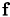is hereby provided in the form of a TBEMFunction, or, to be precise a derived class where the method eval has to be overloaded:

class TMyRHS : public TBEMFunction< complex >
{
public:
virtual complex
eval ( const T3Point & pos,
const T3Point & ) const
{
return sin( pos.x() ) * cos( pos.y() );
}
};

In both cases, the quadrature formula and the BEM function, the value type complex and the function space (for TQuadBEMRHS) are defined as template arguments.

To bring the RHS into the 𝓗-ordering, the vector has to be permuted:

ct2->perm_e2i()->permute( rhs.get() );

# Solving the Discretised System

As standard iteration schemes will usually fail with the above equation system, 𝓗-LU preconditioning is used to ensure and to speed up convergence.

auto B = A->copy();
auto A_inv = factorise_inv( B.get(), acc );

Since the matrix is modified during LU factorisation, a copy of it has to be created and provided for factorisation. The result of factorise_inv is a linear operator suitable for evaluation of the inverse ofand can be used for preconditioning:

TSolver::TInfo solve_info;
auto x = A->col_vector();
solve( A.get(), x.get(), rhs.get(), A_inv.get(), & solve_info );

Upon exit, x contains the computed solution to the initial discrete problem.

The ordering of the unknowns in the solution vector follows the 𝓗-ordering. To bring it back into the original ordering in the grid, use:

ct1->perm_i2e()->permute( x.get() );

The standard finalisation and catch block finishes the example:

DONE();
}
catch ( Error & e )
{
cout << e.to_string() << endl;
}
return 0;
}

# The Plain Program

#include <iostream>
#include <hlib.hh>
using namespace HLIB;
using namespace std;
class TMyRHS : public TBEMFunction< complex >
{
public:
virtual complex
eval ( const T3Point & pos,
const T3Point & ) const
{
return sin( pos.x() ) * cos( pos.y() );
}
};
int main ( int argc, char ** argv )
{
try
{
INIT();
auto grid = read_grid( "grid.tri" );
auto ansatz_fnspace = make_unique< TLinearFnSpace >( grid.get() );
auto test_fnspace = make_unique< TLinearFnSpace >( grid.get() );
auto coord1 = ansatz_fnspace->build_coord();
auto coord2 = test_fnspace->build_coord();
TAutoBSPPartStrat part_strat;
TBSPCTBuilder ct_builder( & part_strat );
auto ct1 = ct_builder.build( coord1.get() );
auto ct2 = ct_builder.build( coord2.get() );
complex kappa( -2, 1 );
THiLoFreqGeomAdmCond adm_cond( kappa, 10 );
TBCBuilder bct_builder;
auto bct = bct_builder.build( ct1.get(), ct2.get(), & adm_cond );
slpbf_t slpbf( kappa, ansatz_fnspace.get(), test_fnspace.get() );
TBFCoeffFn< slpbf_t > coeff_fn( & slpbf, bct->row_ct()->perm_i2e(), bct->col_ct()->perm_i2e() );
TACAPlus< complex > aca( & coeff_fn );
TTruncAcc acc( 1e-4, 0.0 );
TDenseMBuilder< complex > h_builder( & coeff_fn, & aca );
auto A = h_builder.build( bct.get(), acc );
TMyRHS rhsfn;
TQuadBEMRHS< TLinearFnSpace, complex >
rhs_build( 4 );
auto rhs = rhs_build.build( test_fnspace.get(), & rhsfn );
ct2->perm_e2i()->permute( rhs.get() );
auto B = A->copy();
auto A_inv = factorise_inv( B.get(), acc );
TSolver::TInfo solve_info;
auto x = A->col_vector();
solve( A.get(), x.get(), rhs.get(), A_inv.get(), & solve_info );
ct1->perm_i2e()->permute( x.get() );
DONE();
}
catch ( Error & e )
{
cout << e.to_string() << endl;
}
return 0;
}